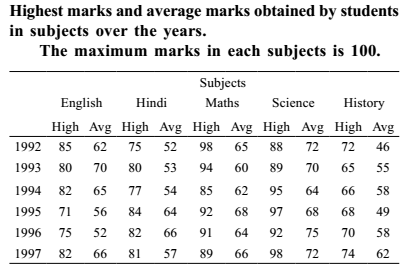## Table chart

#### Data Interpretation

Direction: Read the following Table carefully and answer the questions given below.1. What was the approximate percentage increase in average marks in History from 1992 and 1993?

1.  Percentage increase = average marks in History from 1993 - average marks in History from 1992 X 100 average marks in History from 1992

##### Correct Option: A

 Percentage increase = average marks in History from 1993 - average marks in History from 1992 X 100 average marks in History from 1992

 Percentage increase = 55 - 46 X 100 ≈ 20% 46

1. The highest marks in Hindi in 1993 was what % of the average marks in Mathematics in 1996?

1.  Required Percentage = highest marks in Hindi in 1993 X 100 Average marks in Mathematics in 1996

##### Correct Option: B

 Required Percentage = highest marks in Hindi in 1993 X 100 Average marks in Mathematics in 1996

 Required Percentage = 80 X 100 = 125% 64

1. The difference between the highest marks in Science was maximum between which of the following pairs of years among the given years?

1. The difference between the highest marks in Science in two years = Highest marks in Science in one year - Highest marks in Science in another year
Solve all option one by one

##### Correct Option: D

The difference between the highest marks in Science in two years = Highest marks in Science in one year - Highest marks in Science in another year
Solve all option one by one
Option ( A ) 1992 and 993

The difference between highest marks in Science 1992 and 993 = 89 - 88 = 1
Option ( B ) 1992 and 1996
The difference between highest marks in Science 1992 and 1996 = 92 - 88 = 4
Option ( C ) 1996 and 997
The difference between highest marks in Science 1996 and 1997 = 98 - 92 = 6
Option ( D ) 1992 and 993
The difference between highest marks in Science 1992 and 1995 = 97 - 88 = 9

The maximum difference is in the years 1992 and 1995, since the least value is in 1992 and the highest value is in 1995.

1. What was the grand average marks of the five subjects in 1996?

1.  Grand Average for 1996 = Average marks in ( English + Hindi + Math + Science + History ) 5

##### Correct Option: A

 Grand Average for 1996 = Average marks in ( English + Hindi + Math + Science + History ) 5

 Grand Average = 52 + 66 + 64 + 75 + 58 = 315 = 63 5 5

1. The average highest marks in English in 1992, 1993 and 1996 was exactly equal to the highest marks in Hindi in which of the following years?

1.  Average highest marks in English = Highest marks in English in ( 1992 + 1993 + 1996 ) 3

##### Correct Option: E

 Average highest marks in English = Highest marks in English in ( 1992 + 1993 + 1996 ) 3

 Average highest marks = 85 + 80 + 75 = 240 = 80 3 3